# Classifying Solids

Solid figures are classified into prisms, pyramids, cylinders, spheres and so on.

A prism is a solid that has two congruent bases which are parallel faces. The other faces of the prism are in the shape of rectangles. They are called lateral faces. A prism is named after the shape of its base.

The following diagrams show a triangular prism and a rectangular prism.

A right prism is a prism that has its bases perpendicular to its lateral surfaces.

A Rectangular prism is a prism that has rectangles as its bases. A shoe box, a soap box, a book, etc., are examples of rectangular prism.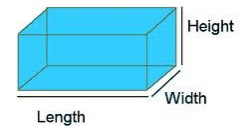A Cube is a special case of a rectangular prism where all faces and bases are congruent squares.

A dice, ice cubes, Rubik’s cube and so on are real world examples of cube shape.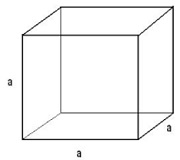A triangular prism is a prism that has triangles as its bases.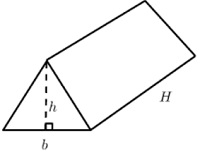Prisms are named after the shape of their bases. A pentagonal prism has pentagons as its bases, a hexagonal prism has hexagons as its bases and so on.

## Cylinder

A Cylinder is a closed solid figure having two parallel circular bases connected by a rectangular curved face.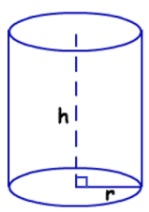A Sphere is solid round figure where every point on its surface is equidistant from its centre.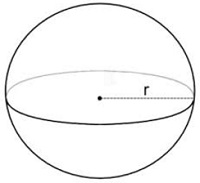A Cone is a closed solid figure whose circular base tapers into a point called apex.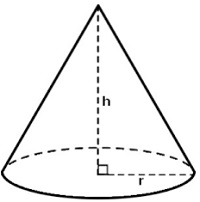## Pyramids

A pyramid is a solid figure that has a base, which can be any polygon, and three or more triangular faces that meet at a point called the apex. These triangular sides are called the lateral faces.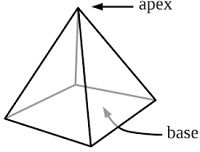Use the diagram to identify the name of the following shape.### Solution

Step 1:

The given figure is a prism whose bases are congruent rectangles.

Step 2:

The given figure is a rectangular prism.

Use the diagram to identify the name of the following shape.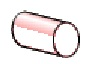### Solution

Step 1:

The given figure is a solid figure whose bases are congruent circles.

Step 2:

The given figure is a cylinder